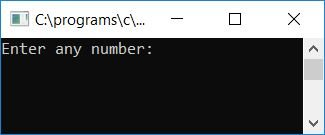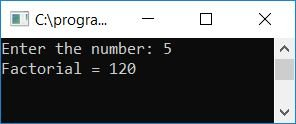# C Program to Find the Factorial of a Number

In this article, we will learn about how to find and print the factorial of any given number, both with and without using a function. Here we have also created a recursive function to find out the factorial of any number. Here is the list of programs you will go through:

But before starting these programs, let's understand how the factorial of a number gets calculated.

### How to find the factor of any number

To find the factorial f of any number, say n, use the formula

`Factorial of n (n!) = (n)*(n-1)*(n-2)*....*3*2*1`

Or

`n! = 1*2*3*....*(n-2)*(n-1)*n`

For example, find the factorial of 5 using the above formula.

```5! = 5*4*3*2*1
= 120```

So the factorial of 5 is 120. Now let's move on and implement it in a C program.

## In C, find the Factorial of a given number

To find the factorial of any given number in C programming, you have to ask the user to enter the number. Now find the factorial of that number using the above formula, as shown in the program given below. As you can see from the formula, if a number is n, then multiplication occurs n times.

For example, if the number is 5, multiplication occurs 5 times, resulting in 5*4*3*2*1. So, in this case, a loop that runs n times is required.

```#include<stdio.h>
#include<conio.h>
int main()
{
int num, i, fact=1;
printf("Enter any number: ");
scanf("%d", &num);
for(i=num; i>0; i--)
fact = fact*i;
printf("\nFactorial of %d = %d", num, fact);
getch();
return 0;
}```

Because the program was written in the Code::Blocks IDE, you will see the following output after a successful build and run:Now supply any number, say 5, and press the ENTER key to see the factorial of 5 as output, as shown here:#### Program Explained

• Get the number as input, say 5, using the scanf() function.
• Create a for loop starting from the number, say 5, in this case.
• Continue running the for loop until the number (here, variable i holds the value of num) becomes 0.
• If the user enters the number 5, the first time the for loop runs, i holds 5 which is greater than 0, and fact holds 1 as its initial value, so fact*i or 5*1 or 5 is assigned to the fact variable.
• At the second run, i is decremented and holds the value 4, which is greater than 0, so fact*i, or 5*4 or 20 is initialized to fact.In the same way, at the third, fourth, and fifth runs, fact holds its final value as 5*4*3*2*1, or 120. At the sixth time, when i gets decremented and becomes 0, which is not greater than 0, the condition evaluates to false, therefore the program flow goes out of the for loop.
• Now print the value of the fact variable that contains the factorial value, say 120, of the given number, say 5.

## Using a Function, find the Factorial of a Number

This program does the same job, but using the user-defined function findFact().

```#include<stdio.h>
#include<conio.h>
int findFact(int);
int main()
{
int num, fact;
printf("Enter any number: ");
scanf("%d", &num);
fact = findFact(num);
printf("\nFactorial of %d = %d", num, fact);
getch();
return 0;
}
int findFact(int n)
{
int i=n, f=1;
while(i>0)
{
f = f*i;
i--;
}
return f;
}```

## Using Recursion, find the Factorial of a Number

Here is another program that will calculate the factorial of any given number using a recursive function:

```#include<stdio.h>
#include<conio.h>
int findFact(int);
int main()
{
int num, fact;
printf("Enter the number: ");
scanf("%d", &num);
fact = findFact(num);
printf("Factorial = %d", fact);
getch();
return 0;
}
int findFact(int val)
{
if(val==1)
return val;
else
return val*findFact(val-1);
}```

Here is the final snapshot of the sample run:#### Program Explained

• Declare the function with one argument to pass the user-supplied value or number.
• Inside the main function, receive the number as input and call the function in such a way that the function will initialize its returning value to the variable that contains the factorial value of the given number, say fact.
• In the recursive function definition, we have checked whether the value or number (passed through argument) is equal to 1 or not; if it is, then return the value of variable val; otherwise, return val*findFact(val-1).
• For example, if the user has supplied 5 as input, then 5 is the argument that gets passed to the recursive function. At first, 5 is not equal to 1, therefore the program flow goes to the else block's statement, and here the returning value is 5*findFact(5-1) or 5*findFact(4). As we have returned the function itself, the function findFact() gets called itself (that is called a recursive function).
• The function is called with argument 4, therefore again 4 is not equal to 1, and again the program flow goes to the else block's statement, therefore the return value will be this time 5*4*findFact(4-3) or 20*findFact(3).
• In the same way, the final return value before the argument value becomes 1 will be returned as 120*findFact(2-1) or 120*findFact(1).
• At last, when the argument value is 1, the program flow goes to the if block's statement, which returns only the value of the variable val that holds the factorial value, say 120, of the number that was passed at the first time as an argument to the function, say 5 here.
• Finally, 120 is initialized to the variable fact inside the main() function.
• Print the value of the fact variable that contains the factorial value of the given number.

## Find the Factorial of All Numbers in a Range

Now this is the last program that will find the factorial of all numbers in a given range. For example, if the user enters 1 and 10 as two numbers (the range), Then the program will find and print the factorial of all numbers from 1 to 10.

```#include<stdio.h>
#include<conio.h>
int findFact(int);
int main()
{
int num1, num2, i;
long int fact;
printf("Enter Range: ");
scanf("%d %d", &num1, &num2);
if(num1<num2)
{
for(i=num1; i<=num2; i++)
{
fact = findFact(i);
printf("\nFactorial of %d = %ld", i, fact);
}
}
else
{
for(i=num2; i<=num1; i++)
{
fact = findFact(i);
printf("\nFactorial of %d = %ld", i, fact);
}
}
getch();
return 0;
}
int findFact(int n)
{
int i=n;
long int f=1;
while(i>0)
{
f = f*i;
i--;
}
return f;
}```

Here is its sample run:#### The same program in different languages

C Quiz

« Previous Program Next Program »